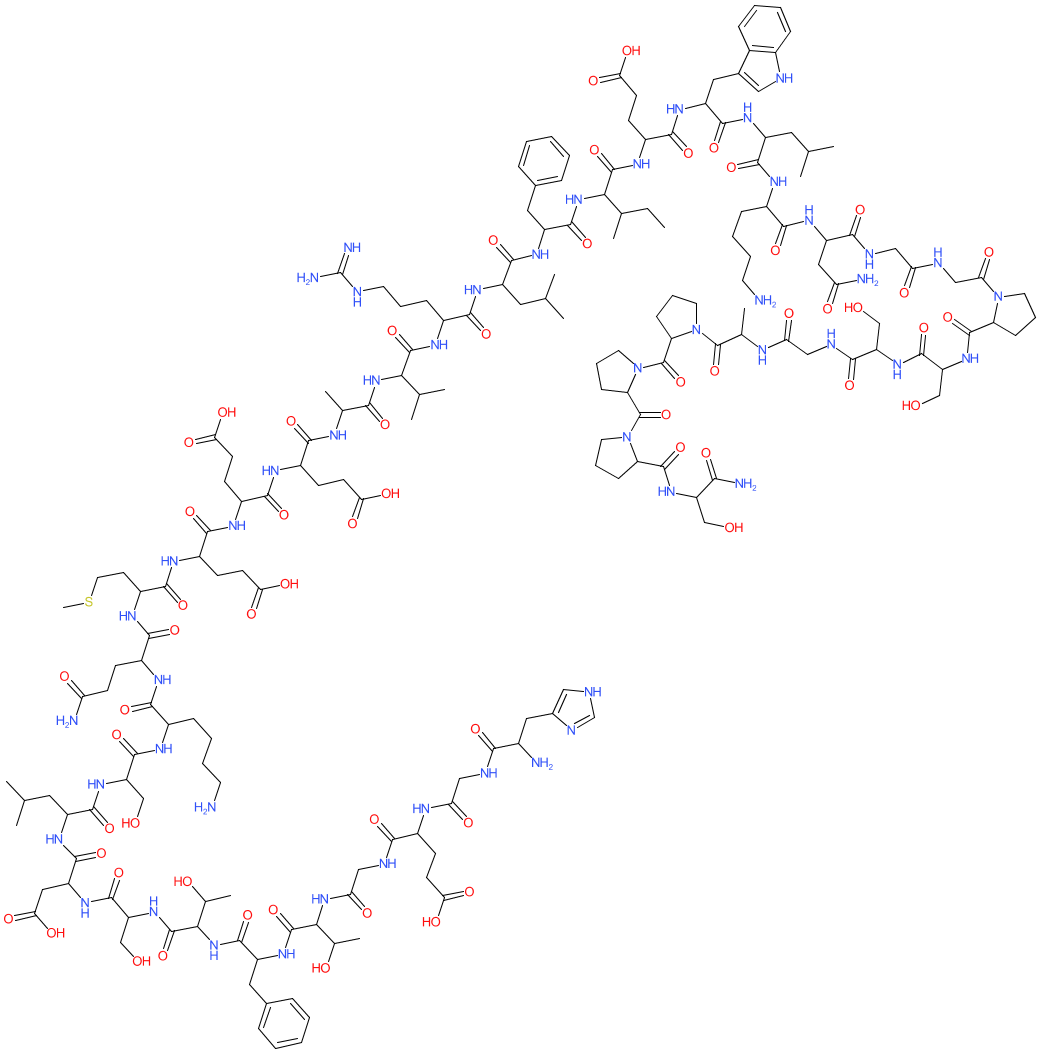GtoPdb Ligand ID: 1135

Synonyms: AC 2993 | AC002993 | AC2993A | Bydureon® | Byetta®
exendin-4 is an approved drug (FDA (2005), EMA (2006))
Comment: This peptide is found in the venom of the Gila monster Heloderma suspectum. Exenatide is the synthetic form of the peptide used in clinical practice. Exendin-4 is a GLP-1 analogue/mimetic . There is some ambiguity surrounding the exact stereochemistry of exendin-4. Representations of the peptide's full chemical structure can be accessed using the PubChem, ChEMBL and ChEBI links that we provide in the Database Links table.Peptide SequenceHGEGTFTSDLSKQMEEEAVRLFIEWLKNGGPSSGAPPPS H-DL-His-Gly-DL-Glu-Gly-DL-xiThr-DL-Phe-DL-xiThr-DL-Ser-DL-Asp-DL-Leu-DL-Ser-DL-Lys-DL-Gln-DL-Met-DL-Glu-DL-Glu-DL-Glu-DL-Ala-DL-Val-DL-Arg-DL-Leu-DL-Phe-DL-xiIle-DL-Glu-DL-Trp-DL-Leu-DL-Lys-DL-Asn-Gly-Gly-DL-Pro-DL-Ser-DL-Ser-Gly-DL-Ala-DL-Pro-DL-Pro-DL-Pro-DL-Ser-NH2
 HELM NotationPEPTIDE1{(H,[dH]).G.(E,[dE]).G.[*C(=O)C(C(C)O)N* |\$_R2;;;;;;;;_R1\$|].(F,[dF]).[*C(=O)C(C(C)O)N* |\$_R2;;;;;;;;_R1\$|].(S,[dS]).(D,[dD]).(L,[dL]).(S,[dS]).(K,[dK]).(Q,[dQ]).(M,[dM]).(E,[dE]).(E,[dE]).(E,[dE]).(A,[dA]).(V,[dV]).(R,[dR]).(L,[dL]).(F,[dF]).[*C(=O)C(C(C)CC)N* |\$_R2;;;;;;;;;_R1\$|].(E,[dE]).(W,[dW]).(L,[dL]).(K,[dK]).(N,[dN]).G.G.(P,[dP]).(S,[dS]).(S,[dS]).G.(A,[dA]).(P,[dP]).(P,[dP]).(P,[dP]).(S,[dS]).[am]}\$\$\$\$V2.0
 Download 2D StructureCanonical SMILES Download Isomeric SMILES Download InChI standard identifier Download InChI standard key Download